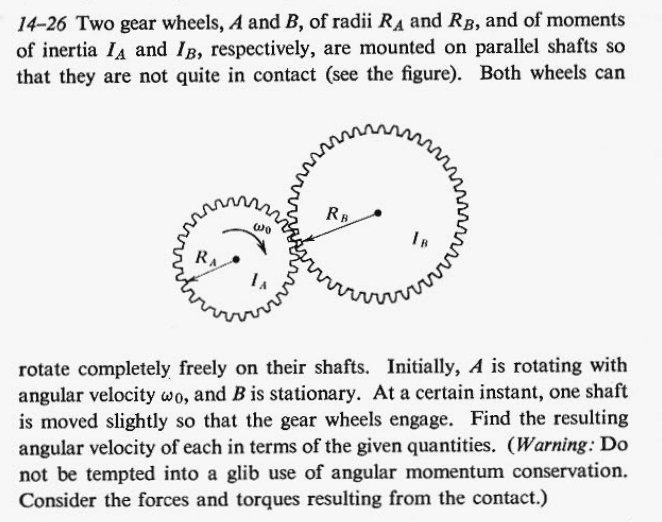# Problem with gears — One geared wheel spins up another

LCSphysicist
Homework Statement:
I am trying to solve a problem by torques and conversation of energy, but the answers don't match.
Relevant Equations:
t = rf
E = constantBy third Newton's law, i would imagine that fa = -fb, so
and assuming f to be the average value

torque in A
-ra*f*t = Waf - Wao
torque in b
rb*f*t = Wbf

and

Ia*wo² = Ib*wbf² + Ia*waf²

it Looks right to you? I really appreciate this method that i thought, but the answers don't match, and i don't know what is wrong.

THe justification to use the conservation of mechanic would be: The work done by one force will be equal to the work done by the reaction force, but opposite in signal, so it cancel.

Homework Helper
Gold Member
You could treat gear A as a flywheel.
There are many industrial presses and shear machines that use that principle for conforming and cutting metal.

•